# DAV Class 3 Maths Chapter 11 Brain Teasers Solutions

The DAV Maths Book Class 3 Solutions and DAV Class 3 Maths Chapter 11 Brain Teasers Solutions of Fractions offer comprehensive answers to textbook questions.

## DAV Class 3 Maths Ch 11 Brain Teasers Solutions

Question 1.

(a) Fraction of the shaded part of the given figure is ______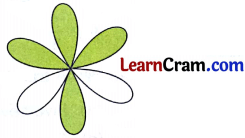(i) $$\frac{2}{6}$$
(ii) $$\frac{4}{6}$$
(iii) $$\frac{5}{6}$$
(iv) $$\frac{3}{6}$$
Solution:
(ii) $$\frac{4}{6}$$

(b) Which of the following sets of figure show $$\frac{1}{6}$$ < $$\frac{4}{6}$$ with respect to shaded part?
(i)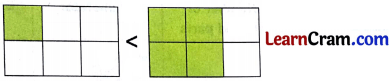(ii)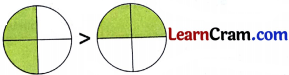(iii)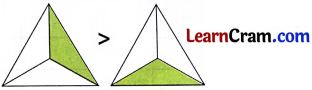(iv)Solution:

(i)(c) Which of the following sets are arranged in ascending order:
(i) $$\frac{10}{5}, \frac{7}{5}, \frac{3}{5}, \frac{2}{5}, \frac{1}{5}$$

(ii) $$\frac{9}{2}, \frac{10}{2}, \frac{7}{2}, \frac{15}{2}, \frac{11}{2}$$

(iii) $$\frac{11}{2}, \frac{7}{2}, \frac{5}{2}, \frac{3}{2}, \frac{10}{2}$$

(iv) $$\frac{1}{5}, \frac{2}{5}, \frac{3}{5}, \frac{7}{5}, \frac{9}{5}$$
Solution:
(iv) $$\frac{1}{5}, \frac{2}{5}, \frac{3}{5}, \frac{7}{5}, \frac{9}{5}$$

(d) In the word ‘MATHEMATICS’. What fraction of letters are vowels?
(i) $$\frac{4}{11}$$
(ii) $$\frac{3}{11}$$
(iii) $$\frac{7}{11}$$
(iv) $$\frac{8}{11}$$
Solution:
(i) $$\frac{4}{11}$$Question 2.
Fill in the blanks.

(a) In $$\frac{9}{10}$$, 9 is called the _______.
Solution:
Numerator

(b) In $$\frac{5}{6}$$, 6 is called the _______.
Solution:
Denominator

(c) Fractions having same denominators are called _______ fractions.
Solution:
Like

Question 3.
Write the fractions in words.

(a) $$\frac{1}{3}$$
Solution:
One-third

(b) $$\frac{1}{9}$$
Solution:
One-ninths

(c) $$\frac{3}{4}$$
Solution:
Three-fourths

(d) $$\frac{1}{8}$$
Solution:
One-eights

(e) $$\frac{5}{6}$$
Solution:
Five-sixths

(f) $$\frac{2}{3}$$
Solution:
Two-third.Question 4.
Write fractions for the following:

(a) Five-sevenths
Solution:
$$\frac{5}{7}$$

(b) Two-tenths
Solution:
$$\frac{2}{10}$$

(c) Five-eights
Solution:
$$\frac{5}{8}$$

(d) Four-ninths
Solution:
$$\frac{4}{9}$$

(e) Three-sevenths
Solution:
$$\frac{3}{7}$$

(f) Four-fifths.
Solution:
$$\frac{4}{5}$$Question 5.
Complete the table given below.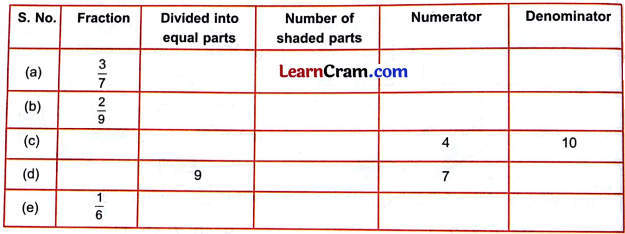Solution: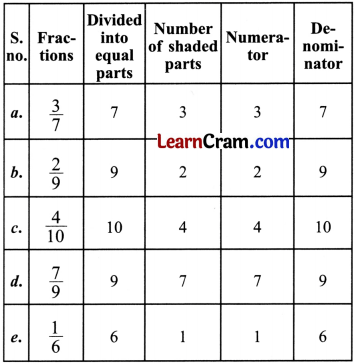Question 6.
Draw figures in your notebook to represent the following fractions:

(a) $$\frac{1}{2}$$
Solution:
$$\frac{1}{2}$$ =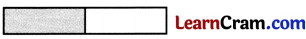(b) $$\frac{1}{6}$$
Solution:
$$\frac{1}{6}$$ =(c) $$\frac{2}{7}$$
Solution:
$$\frac{2}{7}$$ =(d) $$\frac{4}{5}$$
Solution:
$$\frac{4}{5}$$ =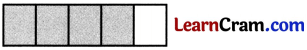Question 7.
Solve the following questions.

(a) $$\frac{9}{10}-\frac{5}{10}$$
Solution:
$$\frac{9}{10}-\frac{5}{10}$$
= $$\frac{9-5}{10}$$
= $$\frac{4}{10}$$

(b) $$\frac{2}{4}+\frac{1}{4}$$
Solution:
$$\frac{2}{4}+\frac{1}{4}$$
= $$\frac{2-1}{4}$$
= $$\frac{1}{4}$$

(c) $$\frac{5}{8}-\frac{2}{8}$$
Solution:
$$\frac{5}{8}-\frac{2}{8}$$
= $$\frac{5-2}{8}$$
= $$\frac{3}{8}$$(d) $$\frac{4}{7}+\frac{1}{7}+\frac{2}{7}$$
Solution:
$$\frac{4}{7}+\frac{1}{7}+\frac{2}{7}$$
= $$\frac{4+1+2}{7}$$
= $$\frac{7}{7}$$

(e) $$\frac{1}{9}+\frac{5}{9}-\frac{2}{9}$$
Solution:
$$\frac{1}{9}+\frac{5}{9}-\frac{2}{9}$$
= $$\frac{1+5-2}{9}$$
= $$\frac{4}{9}$$

(f) $$\frac{8}{11}-\frac{3}{11}$$
Solution:
$$\frac{8}{11}-\frac{3}{11}$$
= $$\frac{8-3}{11}$$
= $$\frac{5}{11}$$

Question 8.
Your are given a fraction: $$\frac{3}{5}$$

(a) Add 2 to numerator and 3 to denominator. What will be the new fraction?
Solution:
(a) Fraction = $$\frac{3}{5}$$
According to the question
$$\frac{3+2}{5+3}=\frac{5}{8}$$ (new fraction)

(b) Multiply numerator by 4 and add 10 to the denominator. What will be the new fraction?
Solution:
$$\frac{3}{5}$$
According to the question
$$\frac{3 \times 4}{5+10}=\frac{12}{15}$$Question 1.
Tick the figures that show halves.

(a)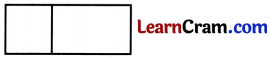(b)(c)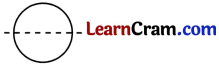(d)Solution:
only
(c)(d)Question 2.
Write ‘T’ for true and ‘F’ for false.

(a)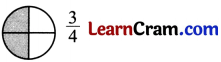Solution:
False

(b)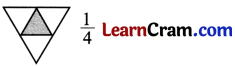Solution:
True

(c)Solution:
False

(d)Solution:
TrueQuestion 3.
Shade to show the two fractions and then write ‘<‘, ‘>‘ or ‘‘

(a)Solution: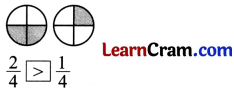(b)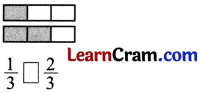Solution: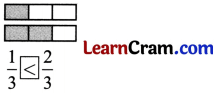(c)Solution: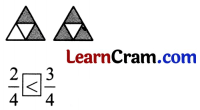(d)Solution:(e)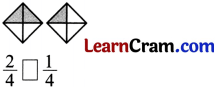Solution:(f)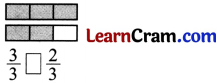Solution:Question 4.

(i) $$\frac{5}{6}+\frac{3}{6}$$
Solution:
$$\frac{5}{6}+\frac{3}{6}$$
= $$\frac{5+3}{6}$$
= $$\frac{8}{6}$$

(ii) $$\frac{8}{2}+\frac{3}{2}$$
Solution:
$$\frac{8}{2}+\frac{3}{2}$$
= $$\frac{8+3}{2}$$
= $$\frac{11}{2}$$

(iii) $$\frac{4}{5}+\frac{3}{5}$$
Solution:
$$\frac{4}{5}+\frac{3}{5}$$
= $$\frac{4+3}{5}$$
= $$\frac{7}{5}$$

(iv) $$\frac{7}{8}+\frac{2}{8}$$
Solution:
$$\frac{7}{8}+\frac{2}{8}$$
= $$\frac{7+2}{8}$$
= $$\frac{9}{8}$$Question 5.
Subtract the following fractions:

(a) $$\frac{8}{6}-\frac{3}{6}$$
Solution:
$$\frac{8}{6}-\frac{3}{6}$$
= $$\frac{8-3}{6}$$
= $$\frac{5}{6}$$

(b) $$\frac{9}{4}-\frac{3}{4}$$
Solution:
$$\frac{9}{4}-\frac{3}{4}$$
= $$\frac{9-3}{4}$$
= $$\frac{6}{4}$$

(c) $$\frac{6}{2}-\frac{3}{2}$$
Solution:
$$\frac{6}{2}-\frac{3}{2}$$
= $$\frac{6-3}{2}$$
= $$\frac{3}{2}$$(d) $$\frac{7}{3}-\frac{4}{3}$$
Solution:
$$\frac{7}{3}-\frac{4}{3}$$
= $$\frac{7-4}{3}$$
= $$\frac{3}{3}$$